# NCERT Solutions for Class 8 Maths Chapter 2 Linear Equations in One Variable Ex 2.2

NCERT Solutions for Class 8 Maths Chapter 2 Linear Equations in One Variable Ex 2.2

### NCERT Solutions for Class 8 Maths Chapter 2 Linear Equations in One Variable Exercise 2.2

Ex 2.2 Class 8 Maths Question 1.Solution:
Let the required number be x.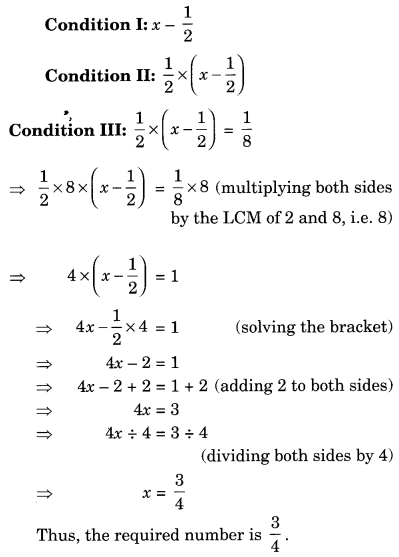Ex 2.2 Class 8 Maths Question 2.
The perimeter of a rectangular swimming pool is 154 m. Its length is 2 m more than twice its breadth. What are the length and the breadth of the pool?
Solution:
Let the breadth of the pool be x m.
Condition I: Length = (2x + 2) m.
Condition II: Perimeter = 154 m.
We know that Perimeter of rectangle = 2 × [length + breadth]
2 × [2x + 2 + x] = 154
⇒ 2 × [3x + 2] = 154
⇒ 6x + 4 = 154 (solving the bracket)
⇒ 6x = 154 – 4 [Transposing 4 from (+) to (-)]
⇒ 6x = 150
⇒ x = 150 ÷ 6 [Transposing 6 from (×) to (÷)]
⇒ x = 25
Thus, the required breadth = 25 m
and the length = 2 × 25 + 2 = 50 + 2 = 52 m.

Ex 2.2 Class 8 Maths Question 3.Solution:
Let the length of each of equal sides of the triangle be x cm.
Perimeter of the triangle = sum of the three sides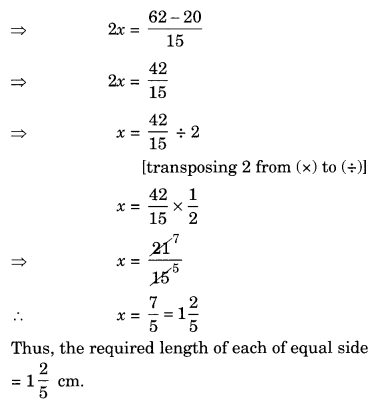Ex 2.2 Class 8 Maths Question 4.
Sum of two numbers be 95. If one exceeds the other by 15, find the numbers.
Solution:
Let one number be x
Other number = x + 15
As per the condition of the question, we get
x + (x + 15) = 95
⇒ x + x + 15 = 95
⇒ 2x + 15 = 95
⇒ 2x = 95 – 15 [transposing 15 from (+) to (-)]
⇒ 2x = 80
⇒ x = 80/2 [transposing 2 from (×) to (÷)]
⇒ x = 40
Other number = 95 – 40 = 55
Thus, the required numbers are 40 and 55.

Ex 2.2 Class 8 Maths Question 5.
Two numbers are in the ratio 5 : 3. If they differ by 18, what are the numbers?
Solution:
Let the two numbers be 5x and 3x.
As per the conditions, we get
5x – 3x = 18
⇒ 2x = 18
⇒ x = 18 ÷ 2 [Transposing 2 from (×) to (÷)]
⇒ x = 9.
Thus, the required numbers are 5 × 9 = 45 and 3 × 9 = 27

Ex 2.2 Class 8 Maths Question 6.
Three consecutive integers add up to 51. What are these integers?
Solution:
Let the three consecutive integers be x, x + 1 and x + 2.
As per the condition, we get
x + (x + 1) + (x + 2) = 51
⇒ x + x + 1 + x + 2 = 51
⇒ 3x + 3 = 51
⇒ 3x = 51 – 3 [transposing 3 to RHS]
⇒ 3x = 48
⇒ x = 48 ÷ 3 [transposing 3 to RHS]
⇒ x = 16
Thus, the required integers are 16, 16 + 1 = 17 and 16 + 2 = 18, i.e., 16, 17 and 18.

Ex 2.2 Class 8 Maths Question 7.
The sum of three consecutive multiples of 8 is 888. Find the multiples.
Solution:
Let the three consecutive multiples of 8 be 8x, 8x + 8 and 8x + 16.
As per the conditions, we get
8x + (8x + 8) + (8x + 16) = 888
⇒ 8x + 8x + 8 + 8x + 16 = 888
⇒ 24x + 24 = 888
⇒ 24x = 888 – 24 (transposing 24 to RHS)
⇒ 24x = 864
⇒ x = 864 ÷ 24 (transposing 24 to RHS)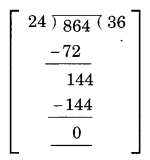⇒ x = 36
Thus, the required multiples are
36 × 8 = 288, 36 × 8 + 8 = 296 and 36 × 8 + 16 = 304,
i.e., 288, 296 and 304.

Ex 2.2 Class 8 Maths Question 8.
Three consecutive integers are such that when they are taken in increasing order and multiplied by 2, 3, and 4 respectively, they add up to 74. Find these numbers.
Solution:
Let the three consecutive integers be x, x + 1 and x + 2.
As per the condition, we have
2x + 3(x + 1) + 4(x + 2) = 74
⇒ 2x + 3x + 3 + 4x + 8 = 74
⇒ 9x + 11 = 74
⇒ 9x = 74 – 11 (transposing 11 to RHS)
⇒ 9x = 63
⇒ x = 63 ÷ 9
⇒ x = 7 (transposing 7 to RHS)
Thus, the required numbers are 7, 7 + 1 = 8 and 7 + 2 = 9, i.e., 7, 8 and 9.

Ex 2.2 Class 8 Maths Question 9.
The ages of Rahul and Haroon are in the ratio 5 : 7. Four years later the sum of their ages will be 56 years. What are their present ages?
Solution:
Let the present ages of Rahul and Haroon he 5x years and 7x years respectively.
4 years later, the age of Rahul will be (5x + 4) years.
4 years later, the age of Haroon will be (7x + 4) years.
As per the conditions, we get
(5x + 4) + (7x + 4) = 56
⇒ 5x + 4 + 7x + 4 = 56
⇒ 12x + 8 = 56
⇒ 12x = 56 – 8 (transposing 8 to RHS)
⇒ 12x = 48
⇒ x = 48 ÷ 12 = 4 (transposing 12 to RHS)
Hence, the required age of Rahul = 5 × 4 = 20 years.
and the required age of Haroon = 7 × 4 = 28 years.

Ex 2.2 Class 8 Maths Question 10.
The number of boys and girls in a class are in the ratio 7 : 5. The number of boys is 8 more than the numbers of girls. What is the total class strength?
Solution:
Let the number of boys be 7x
and the number of girls be 5x
As per the conditions, we get
7x – 5x = 8
⇒ 2x = 8
⇒ x = 8 ÷ 2 = 4 (transposing 2 to RHS)
the required number of boys = 7 × 4 = 28
and the number of girls = 5 × 4 = 20
Hence, total class strength = 28 + 20 = 48

Ex 2.2 Class 8 Maths Question 11.
Baichung’s father is 26 years younger than Baichung’s grandfather and 29 years older than Baichung. The sum of the ages of all the three is 135 years. What is the age of each one of them?
Solution:
Let the age of Baichung be x years.
The age of his father = x + 29 years,
and the age of his grandfather = x + 29 + 26 = (x + 55) years.
As per the conditions, we get
x + x + 29 + x + 55 = 135
⇒ 3x + 84 = 135
⇒ 3x = 135 – 84 (transposing 84 to RHS)
⇒ 3x = 51
⇒ x = 51 ÷ 3 (transposing 3 to RHS)
⇒ x = 17
Hence Baichung’s age = 17 years
Baichung’s father’s age = 17 + 29 = 46 years,
and grand father’s age = 46 + 26 = 72 years.

Ex 2.2 Class 8 Maths Question 12.
Fifteen years from now Ravi’s age will be four times his present age. What is Ravi’s present age?
Solution:
Let the present age of Ravi be x years.
After 15 years, his age will be = (x + 15) years
As per the conditions, we get
⇒ x + 15 = 4x
⇒ 15 = 4x – x (transposing x to RHS)
⇒ 15 = 3x
⇒ 15 ÷ 3 = x (transposing 3 to LHS)
⇒ x = 5
Hence, the present age of Ravi = 5 years.

Ex 2.2 Class 8 Maths Question 13.Solution:
Let the required rational number be x.
As per the condition, we get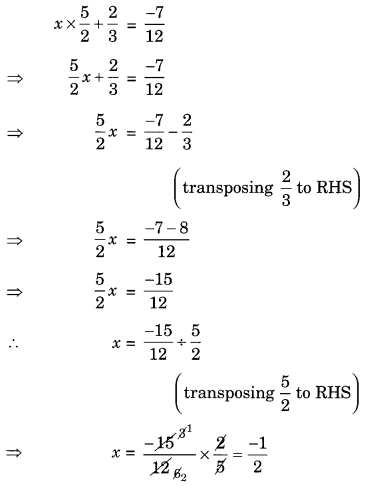Hence, the required rational number is 1/2

Ex 2.2 Class 8 Maths Question 14.
Lakshmi is a cashier in a bank. She has currency notes of denominations ₹ 100, ₹ 50 and ₹ 10, respectively. The ratio of these notes is 2 : 3 : 5. The total cash with Lakshmi is ₹ 4,00,000. How many notes of each denomination does she have?
Solution:
Let the number of ₹ 100, ₹ 50 and ₹ 10 notes be 2x, 3x and 5x respectively.
Converting all the denominations into rupees, we have
2x × 100, 3x × 50 and 5x × 10 i.e. 200x, 150x and 50x
As per the conditions, we have
200x + 150x + 50x = 4,00,000
⇒ 400x = 4,00,000
⇒ x = 4,00,000 ÷ 400 (transposing 400 to RHS)
⇒ x = 1,000
Hence, the required number of notes of
₹ 100 notes = 2 × 1000 = 2000
₹ 50 notes = 3 × 1000 = 3000
and ₹ 10 notes = 5 × 1000 = 5000

Ex 2.2 Class 8 Maths Question 15.
I have a total of ₹ 300 in coins of denomination ₹ 1, ₹ 2 and ₹ 5. The number of ₹ 2 coins is 3 times the number of ₹ 5 coins. The total number of coins is 160. How many coins of each denomination are with me?
Solution:
Let the number of ₹ 5 coins be x.
Number of ₹ 2 coins = 3x
Total number of coins = 160
Number of ₹ 1 coin = 160 – (x + 3x) = 160 – 4x
Converting the number of coins into rupees, we have
x coins of ₹ 5 amount to ₹ 5x
3x coins of ₹ 2 amount to ₹ 3x × 2 = ₹ 6x
and (160 – 4x) coins of ₹ 1 amount to ₹ 1 × (160 – 4x) = ₹ (160 – 4x)
As per the conditions, we have
5x + 6x + 160 – 4x = 300
⇒ 7x + 160 = 300
⇒ 7x = 300 – 160 (transposing 160 to RHS)
⇒ 7x = 140
⇒ x = 140 ÷ 7 (transposing 7 to RHS)
⇒ x = 20
Thus, number of ₹ 5 coins = 20
Number of ₹ 2 coins = 3 × 20 = 60
and Number of ₹ 1 coins = 160 – 4 × 20 = 160 – 80 = 80

Ex 2.2 Class 8 Maths Question 16.
The organisers of an essay competition decide that a winner in the competition gets a prize of ₹ 100 and a participant who does not win gets a prize of ₹ 25. The total prize money distributed is ₹ 3,000. Find the number of winners, if the total number of participants is 63.
Solution:
Let the number of winners = x
Number of participants who does not win the prize = (63 – x)
Amount got by winners = ₹ 100 × x = ₹ 100x
Amount got by loosers = ₹ (63 – x) × 25 = ₹ (1575 – 25x)
As per the conditions, we get
100x + 1575 – 25x = 3000
⇒ 75x + 1575 = 3000
⇒ 75x = 3000 – 1575 (transposing 1575 to RHS)
⇒ 75x = 1425
⇒ x = 1425 ÷ 75 (Transposing 75 to RHS)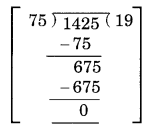⇒ x = 19
Thus, the number of winners = 19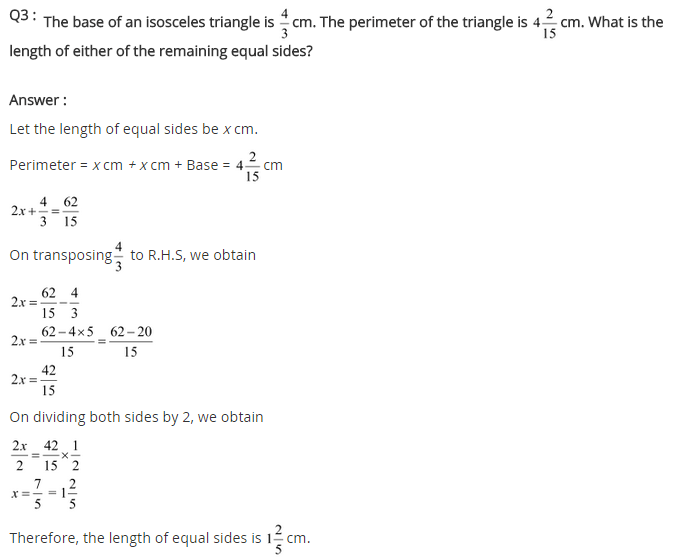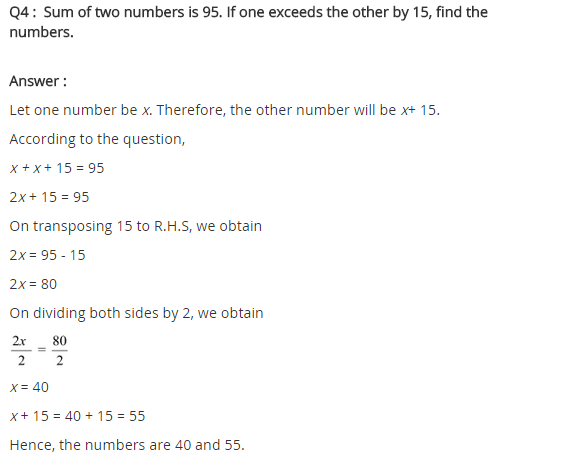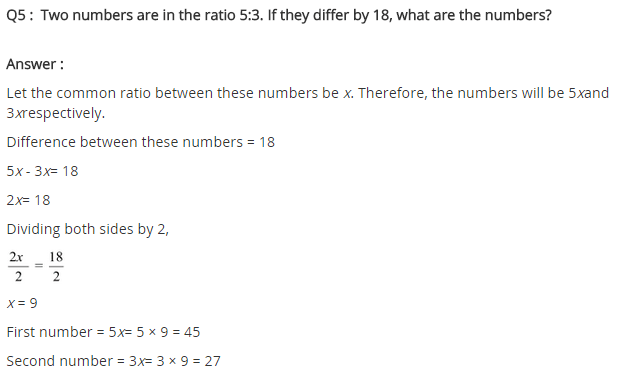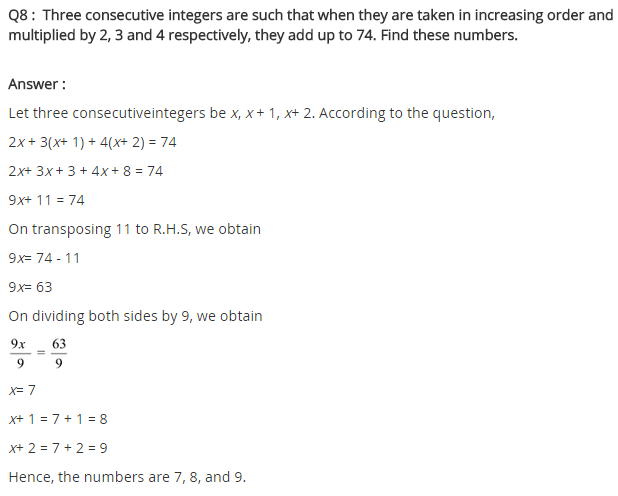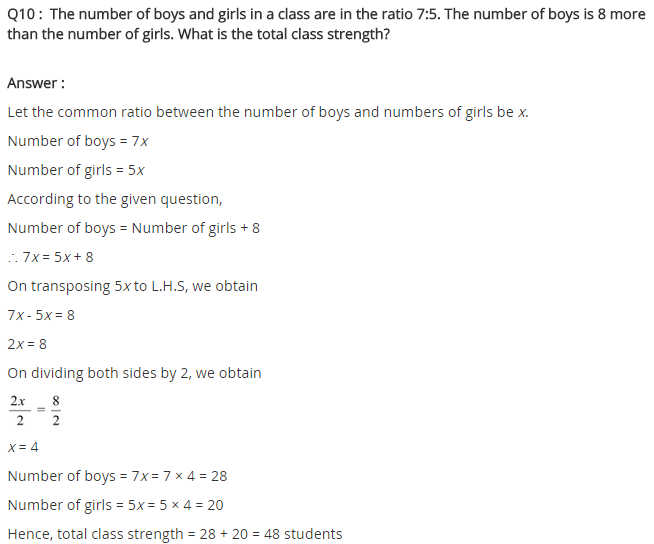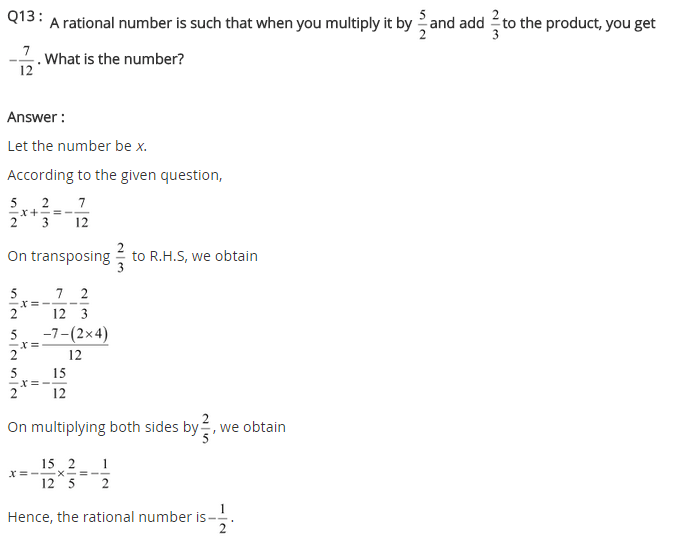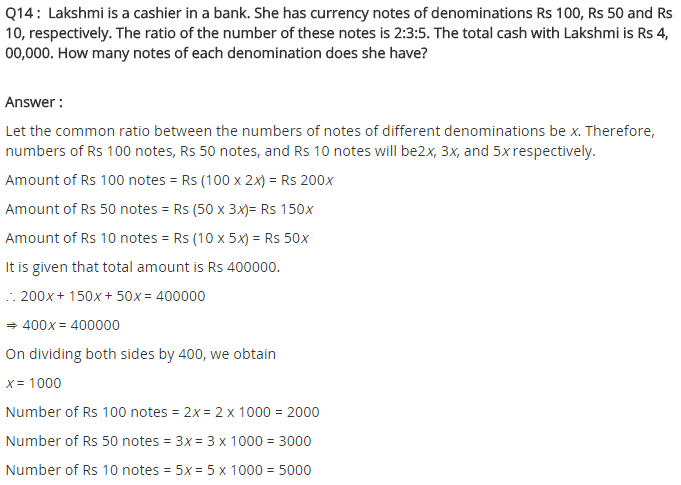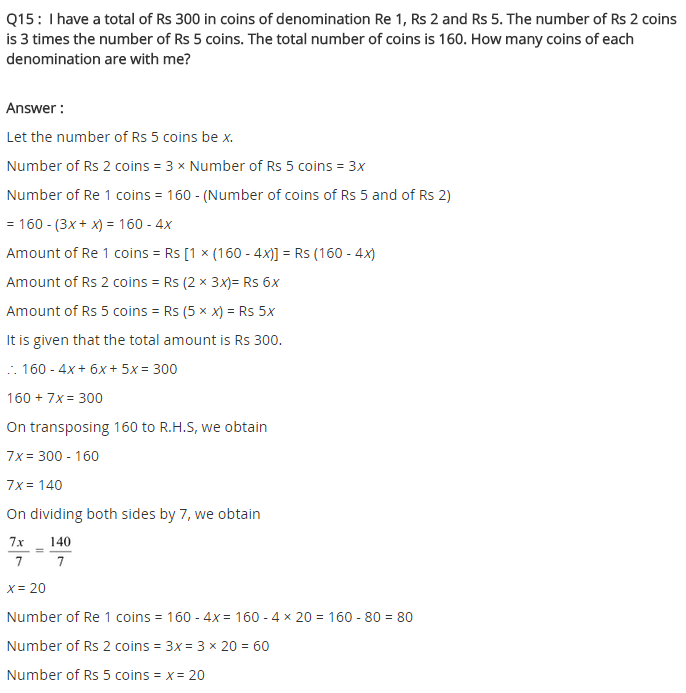## SabDekho

The Complete Educational Website